# Chapter 4 – Waves

From ‘Highways in the Coastal Environment: Second Edition’ by FHWA

#### Chapter 4 – Waves

4.1 – 4.2 – 4.3 – 4.4 – 4.5 – 4.6

Waves cause some of the primary hydraulic forces in coastal engineering applications. Water waves are caused by a disturbance of the water surface. The original disturbance may be caused by winds, boats or ships (wakes), or other disturbances such as underwater landslides due to earthquakes (tsunamis). Most waves are generated by wind. After waves are formed, they can propagate across the surface of the sea for thousands of miles. When waves break on a shoreline or coastal structure, they have fluid velocities and accelerations that can impart significant forces.

Practical wave mechanics is a blend of theories and empirical evidence. Several wave theories including the small-amplitude wave theory and Stokes 2nd order wave theory developed in the late 1800’s are still used today. Much of the practical scientific study of coastal waves changed during World War II. Plans for amphibious landings such as at Normandy on D-Day and on the Pacific Islands later in the war required as good a prediction as possible of the surf conditions that the landing craft could expect. Research led to equations for forecasting wave heights based on wind speeds as well as equations for estimating how waves break in shallow water. That research revolutionized nearshore oceanography and led to predecessors of the coastal engineering tools still used today and summarized briefly below.

#### 4.1 Definitions, Theories, and Properties of Waves

This section introduces the basic definitions used in wave mechanics, very briefly introduces several of the most important wave theories, and presents some of the more useful engineering properties of waves. Many engineering applications of wave theories rely on the small-amplitude wave theory. However, there are several important engineering properties that can only be explained by more complex theories or by empirical methods.

Figure 4.1 depicts the basic parameter definitions in the simplest model of water waves. The wave in Figure 4.1 is assumed to be progressing toward the right. The individual waves are assumed to be long-crested (such that the 2-dimensional plane shown in Figure 4.1 is sufficient) and part of an infinite train of repeating waves. The basic length scales used to define the wave are the wavelength (L) defined as the distance between wave crests, and the wave height (H) defined as the difference between the elevation of the crest and the trough of an individual wave. Waves are called monochromatic waves in this simplest model since the waves are all the same wavelength. The water depth (d) is defined as measured to the still water level (SWL), the level of the water if the waves were not present. Wave period (T) is the time required for a wave to travel one wavelength.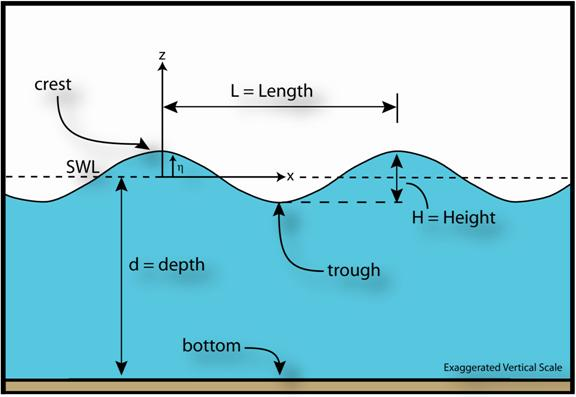Figure 4.1. Wave parameter definitions.

Small amplitude wave theory provides estimates of many of the basic engineering properties of the monochromatic wave train on a fixed water depth. The result is a progressive, monochromatic wave solution to the boundary value problem consisting of the governing equations of motion for irrotational motion of an inviscid fluid (Laplace’s Equation) and the appropriate boundary conditions.

A fundamental assumption in the theoretical development of the theory is that the wave amplitude is small. Amplitude is defined as a=H/2. The small-amplitude wave theory is often called “linear wave theory” because the small-amplitude assumption allows for the boundary conditions to be mathematically “linearized” and thus solved.

In spite of the seemingly limiting assumption of small waves, many of the basic properties of waves are well estimated by small-amplitude theory. For more information on the theoretical basis and results of small-amplitude wave theory, see Dean and Dalrymple (1991) or Sorensen (1993).

The primary small-amplitude theory solution for the water surface elevation, η, is a cosine wave (as shown in Figure 4.1) described by Equation 4.1.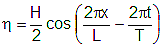(4.1)

where:

 η = water surface elevation (as measured from the SWL) H = wave height x = horizontal position t = Time L = Wavelength T = wave period

Small-amplitude wave theory indicates that three of the four basic parameters describing the basic wave model are not independent. Specifically, the wavelength, L, is a function of water depth and wave period, T (Equation 4.2):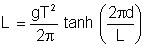(4.2)

where:

 g = acceleration due to gravity d = water depth tanh = hyberbolic tangent function

In deepwater, where the depth is greater than one-half the wavelength (d>L/2), Equation 4.2 reduces to: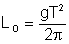(4.3)

where:

 LO = wavelength in deepwater

Wave speed, or celerity, is the speed at which the wave form moves across the ocean surface. Based on the parameters above, this is: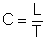(4.4)

where:

 C = wave celerity

In deepwater, Equation 4.4 becomes: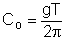(4.5)

where:

 CO = wave celerity in deep water

Note that Equation 4.5 suggests that waves of different periods move at different speeds in deep water.

In shallow water, where depth is less than one-twenty-fifth of the wavelength (i.e., d < L/25), Equation 4.4 becomes: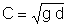(4.6)

Equation 4.6 indicates that all waves move at the same speed in shallow water regardless of wave period and that waves slow down as they move into shallower water.

Equation 4.2 is an implicit equation for L. One explicit approximation to Equation 4.2 is Eckart’s approximation: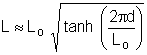(4.7)

Equation 4.7 gives results within 5% of those from Equation 4.2. Given the lack of precision of input conditions in many coastal design situations as well as the uncertainty inherent in the analytical methods this accuracy is often acceptable for engineering purposes.

Instantaneous water particle velocities in waves are given by the small-amplitude theory as: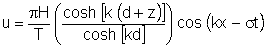(4.8)

and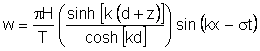(4.9)

where:

 u = horizontal component of water particle velocity w = vertical component of water particle velocity k = wave number = 2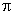/L σ = wave frequency = 2/T z = vertical direction (measured from the SWL, see Figure 4.1) cosh = hyperbolic cosine function sinh = hyperbolic sine function

Note that the velocity field in waves is oscillatory with respect to the wave phase or the position of the wave crest. There are essentially three parts to the velocity equations: (1) an oscillatory term with the sine or cosine function, (2) a hyperbolic function of z which is an exponential decrease in velocity with distance below the free surface, and (3) a magnitude term,H/T.

Maximum velocities occur when the phase is such that the sine or cosine term equals 1.0, e.g. when cos(kx-σt)=1 for Equation 4.8. Considering the vertical variation in velocity, maximum velocity occurs at the free surface (z=0).

Note that, based on the assumptions inherent in the small amplitude theory, the free-surface is taken as z=0 instead of at some higher elevation such as z=η. The maximum forward water particle velocity occurs on the free surface of the crest of the wave and is: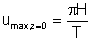(4.10)

The wave-induced horizontal velocity on the bottom (z=-d), which can control sediment movement on the bottom, becomes: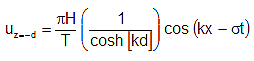(4.11)

with a maximum value where cos(kx-σt)=1 of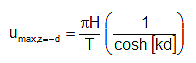(4.12)

The instantaneous water particle accelerations in a wave field are given by: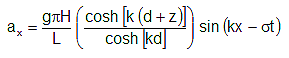(4.13)

and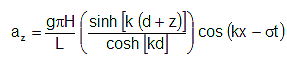(4.14)

where:

 ax = horizontal component of water particle acceleration. az = vertical component of water particle acceleration.

Water particle displacements or the paths of individual water particles in water waves can be estimated by small-amplitude wave theory. In deepwater the paths are circular with the magnitude of the circular motion decreasing with distance below the free surface (Figure 4.2). At a depth of about one-half the wavelength the wave-induced orbital movements die out (see Dean & Dalrymple 1991; Sorensen 1993; or USACE 2002 for the particle equations). Below the depth, d=L/2, no surface wave motion is felt.

Figure 4.3 illustrates how particle paths are elliptical in intermediate and shallow depths as shown in Figure 4.3. The vertical amplitude of the elliptical motion decreases with increasing depth. At the bottom, the water particles move back and forth along the bottom. Scuba divers in shallow water are familiar with this back and forth motion and often refer to it as “surge.” The magnitude of the motion can cause difficult working conditions for divers and the corresponding accelerations can make for nauseous conditions.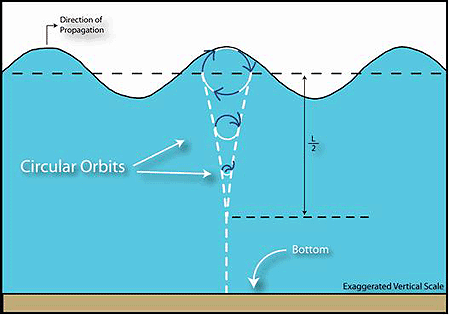Figure 4.2. Water particle paths under waves in deep water.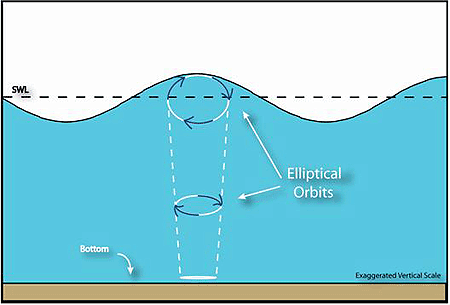Figure 4.3 Water particle paths under waves in shallow and intermediate water depths.

The small-amplitude wave theory provides adequate approximations of the kinematics of wave motion for many engineering applications. However, when waves are very large or in very shallow water, small-amplitude theory results may not be adequate. Higher-order wave theories, such as higher order Stokes wave theories, cnoidal wave theory, and solitary wave theory address these important situations more appropriately. Numerical wave theories, however, have the broadest range of applicability.

Small-amplitude wave theory may not adequately predict the distortion of the water surface profile for large waves or for shallow water waves. The sinusoidal shape of the free surface of a water wave (shown in Figure 4.1) is a reasonable engineering model of the free surface of smaller waves in deepwater.

However, larger waves are known to have water surface profiles that are more like those shown in Figure 4.4. Stokes 2nd order theory predicts water surface profiles that are the sum of two phase-locked sinusoidal waves with the smaller having half the wavelength of the first. The resulting water surface profile has more sharply peaked crests and flatter troughs than the sinusoidal profile from small-amplitude theory.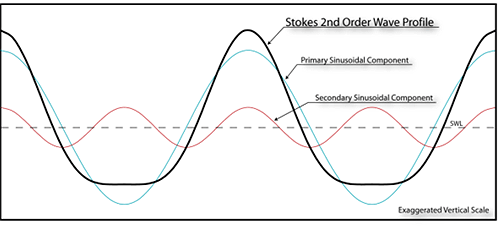Figure 4.4. Stokes 2nd order wave theory water surface profile.

Numerical wave theories can predict water surface shape and kinematics for large waves in deep or shallow water to any level of accuracy. The iterative power of the computer is used to more precisely solve the governing equations and appropriate boundary conditions. The most commonly available numerical wave theories are Dean’s streamfunction wave theory (Dean 1965, Dean 1974) and Rienecker and Fenton’s potential theory (Rienecker and Fenton 1981).

Two shallow water wave theories are the solitary wave theory and the cnoidal wave theory. These are both analytical theories for waves in very shallow water. The solitary wave considers a single wave. The cnoidal wave is part of a train of monochromatic waves.

The phenomenon of a non-sinusoidal shape of the water surface profile can become obvious for swell in shallow water. Cnoidal waves or the numerical theories can model this phenomenon well.

The wave kinematics, including orbital velocities and accelerations, predicted by higher-order wave theories vary from those predicted by the small-amplitude theory. The velocities and accelerations under the crests of the waves will be larger but of shorter duration than those predicted by linear theory. However, the variation from the small-amplitude theory is often less than 20-30%. This can be important for wave loads.

The total wave energy of a wave train is the sum of its kinetic energy and its potential energy. The kinetic energy is that part of the total energy due to water particle velocities associated with the orbital wave-induced motion discussed above. Potential energy is that part of the energy resulting from part of the fluid mass in the wave crest being above the wave trough. The total energy density (energy per unit surface area) in a wave train is given by small-amplitude wave theory as: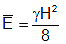(4.15)

where: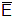= total energy in a wave train per unit area of sea averaged over one wavelength H = wave height g = specific weight of water

The implication of Equation 4.15 is that energy in a sea state is directly related to the square of the wave height. Wave height can be used as a measure of energy in a sea-state. Energy is very sensitive to wave height and doubling the wave height increases the energy in the sea-state four fold.

Waves propagate energy across the sea by moving in wave groups. Interestingly, the groups of waves, and thus the energy in the waves, can move at different speeds than the individual waves. The wave group velocity (Cg), or the velocity at which energy is propagated, is related to the individual wave celerity as: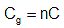(4.16)

where:

 n = ratio of wave group velocity to wave celerity (given by Equation 4.17 below) C = wave celerity (defined by Equation 4.4)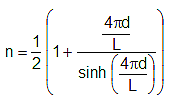(4.17)

The value n varies from ½ to 1. In deepwater, it approaches n = ½. In shallow water, it approaches n = 1. Thus, in deepwater, the wave energy is propagated at about one-half (½) of the individual wave celerity.

However, in shallow water the energy moves at the individual wave celerity: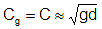(4.18)

The wave power, or wave energy flux in a wave train, is given by: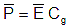(4.19)

where: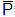= wave power= total wave energy density (defined in Equation 4.15) Cg = wave group velocity (defined in Equation 4.16)

Wave energy flux entering the surf zone has been related to the longshore sediment transport rate, wave setup in the surf zone, and other surf zone dynamics as discussed in Chapter 5.

#### 4.2 Wave Transformation and Breaking

As waves move toward the coast into shallower water depths, they undergo transformations and ultimately, they break. The wave period of individual waves remains constant through the transformations until breaking but the direction of propagation and the wave height can change significantly. Transformations include shoaling, refraction, diffraction, attenuation and reflection. There are different ways that waves break when they hit a shoreline or structure. The concept of a depth-limited wave height in shallow water can be very valuable in some coastal engineering applications.

As a wave moves into shallower water the wavelength decreases (recall Equation 4.2) and the wave height increases. For two-dimensional propagation, i.e. straight toward shore, the increase in wave height can be theoretically shown, by conservation of wave energy considerations, to be: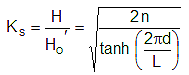(4.20)

where:

 Ks = shoaling coefficient H = wave height Ho‘ = deepwater wave height

The shoaling coefficient increases from Ks = 1.0 up to perhaps as much as Ks = 1.5 as the individual wave moves into shallower water until the wave breaks via the depth-limited mechanism discussed below.

Wave crests bend as they move into shallower water via refraction. As waves approach the beach at an angle, a portion of the wave is in shallower water and moving more slowly than the rest of the wave. Viewed from above (Figure 4.5) the wave crest begins to bend and the direction of wave propagation changes. Refraction changes the height of waves as well as the direction of propagation.

There are two general types of models for monocromatic wave refraction. Wave-ray models, the older type of model, estimate the path of wave rays, lines perpendicular to the wave crests. These wave ray models are based on Snell’s Law. They can provide reasonable estimates of refraction but have problems with crossing wave rays or “caustics.” These are physically impossible since they imply an infinite wave height. Grid-based refraction models solve some form of governing differential equation for the wave height field across arbitrary bottom contours and avoid this “caustic” problem.

Diffraction is the bending of wave crests as they spread out into quieter waters. An example of wave diffraction is the spreading of wave energy around the tip of a breakwater into the lee of the breakwater. The wave crest, as viewed from above, can wrap itself around the tip of the breakwater and appears to be propagating from that tip location into the quieter water. Diffraction also occurs in open water as waves propagate across varying depths. Thus, wave diffraction and refraction often occur together and any separation of the two mechanisms can be problematic in engineering modeling.

The combination of wave refraction and diffraction can cause wave energy to be focused on headlands or reefs and de-focused in embayments as shown in Figure 4.6. Thus wave heights can be increased on headlands and decreased in embayments.

Numerical wave refraction models are often combined with diffraction models. One such combined model is the REF/DIF model originally developed by Kirby and Dalrymple (1983).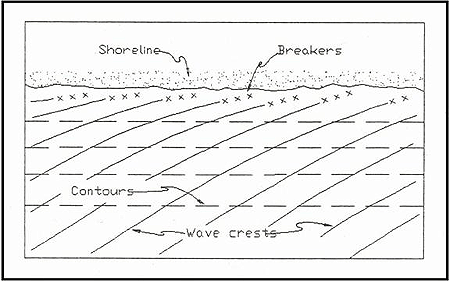Figure 4.5. Bending of wave crests as they approach the shore due to refraction (from USACE 2002).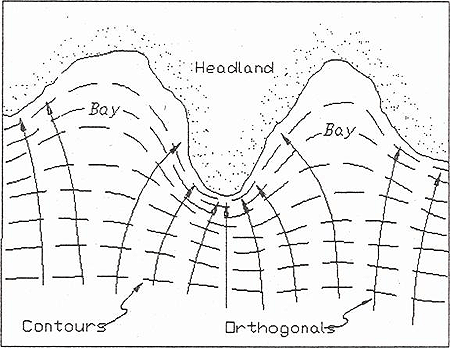Figure 4.6. Wave energy focused on headland by a wave refraction and diffraction (from USACE 2002).

Wave energy has the ability to propagate very long distances across the ocean with very little loss of energy. However, wave height can decrease as a wave propagates across flat bottoms in shallow water. Energy can be lost due to bottom friction and other processes. These energy losses, or attenuation, can significantly reduce heights. Wave breaking across a shallow bar or reef is also sometimes referred to as wave attenuation.

Wave energy is usually partially reflected when it hits a shoreline or structure. The reflection coefficient is defined as the ratio of the incident wave height to the reflected wave height: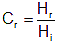(4.21)

where:

 Cr = reflection coefficient Hr = reflected wave height Hi = incident wave height

The reflection coefficient can vary from 0 < Cr < 1 depending on the shoreline or structure type. Smooth, vertical walls have reflection coefficients of 0.9 < Cr < 1.0. Reflection from sloping walls, revetments and beaches is very sensitive to slope and can vary from 0.05 to 0.9 for different smooth slopes. The lower values are for very flat slopes. Typical values of reflection coefficient for sandy beaches and rubble-mound structures are 0 < Cr < 0.45 and 0 < C< 0.55 respectively (USACE 1984).

Waves break at two general limits:

• In deepwater, waves can become too steep and break when the wave steepness defined as, H/L, approaches 1/7.
• In shallow water, waves break when they reach a limiting depth (see Figure 4.7).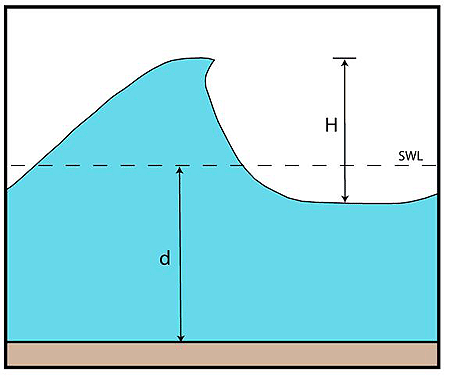Figure 4.7. Depth-limited wave breaking in shallow water.

This depth-limited breaking can be very important in the design of coastal revetments protecting highways. For an individual wave, the limiting depth is roughly equal to the wave height and lies in the practical range: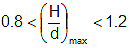(4.22)

where: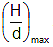= maximum ratio of wave height to water depth.

The variation expressed in Equation 4.22 is due to nearshore slope and incident wave steepness, H/L.

Steeper nearshore slopes result in higher values of.

A practical value when there is a mild sandy slope offshore of the structure is: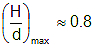(4.23)

Which corresponds with a theoretical limit from solitary wave theory of: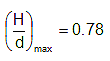The depth-limited wave height can be expressed as: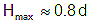(4.24)

where:

 Hmax = maximum wave height d = Depth of water (as shown in Figure 4.7)

Equation 4.24 is often useful in selecting an upper limit for a design wave height for coastal structures in shallow water. Given an estimate of the water depth at the structure location, the maximum wave height Hmax that can exist in that depth of water is known. Any larger waves would have broken farther offshore and been reduced to this Hmax. Equation 4.24 is a nominal limit and is not conservative on sloped bottoms. Note that depth, d, must be the total water depth, including tides and design surge levels, and allowances for scour if applicable.

There are four different types of breaking waves. Typical water surface profiles for these breaker types are shown in Figure 4.8. Breaker type is controlled by wave steepness (H/L), beach or structure slope, and local wind direction.

Spilling and plunging are the most common breaker types on sandy beaches. An example of a plunging wave is shown in Figure 4.9. When waves plunge, the wave form stands up in vertical face that then plunges over often forming a “tube” of the sort that good surfers like. If a breaker plunges immediately offshore of a vertical structure such as a seawall, a pocket of air can be trapped between the water and the structure. The air pocket can be compressed and produce extremely large, short duration loads on the vertical structure.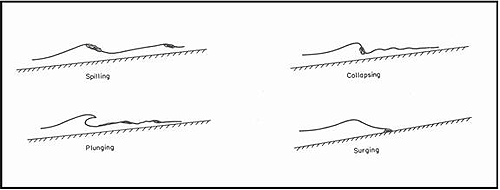Figure 4.8. Wave breaker types (Sorensen 1993).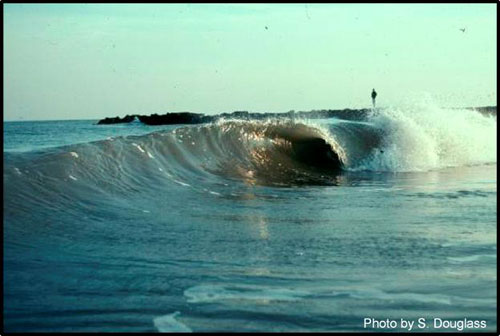Figure 4.9. An example of a plunging breaker (from Douglass 2002).

Surging breakers occur on very steeply sloped beaches and on coastal structures. The surging breaker type is really just a form of wave reflection. The collapsing breaker is intermediate between the plunging and surging types. Collapsing breakers are often the most damaging to coastal structures, particularly rubble-mound structures, because the entire wave front collapses on the structure generating extremely high wave particle velocities and accelerations. Figure 4.10 shows a rock breakwater being struck by a collapsing wave.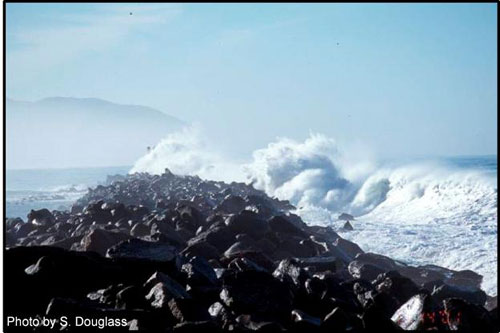Figure 4.10. An example of a “collapsing” breaker (Morro Bay, California).

#### 4.3 Irregular Waves

The smooth water surfaces of monochromatic wave theories are not often realistic representations of the sea state on real ocean or bay surfaces. Figure 4.11 shows a long period swell approaching the Pacific coast. For long-period swell situations such as this one, the monochromatic theories are appropriate. Note in the background that there is a continuous train of waves that are of almost the same height with long, straight wave crests (except where they begin to refract and break). The wave profiles show some of the behavior of sharp crests and flat troughs discussed above for Stokes 2nd order wave and cnoidal wave theories.

Another, more typical, water surface is shown in Figure 4.12. Individual smooth wave trains are not obvious and the sea state in the bay looks much more chaotic and short-crested. Figure 4.13 shows an even more extreme case of an actively growing sea state. This photograph was taken from an offshore platform in the Gulf of Mexico during a tropical storm. The more typical sea-states, like those shown in Figure 4.12 and Figure 4.13, can be referred to as “irregular waves,” or random waves, since they do not have the smooth, repeating shapes of monochromatic theories.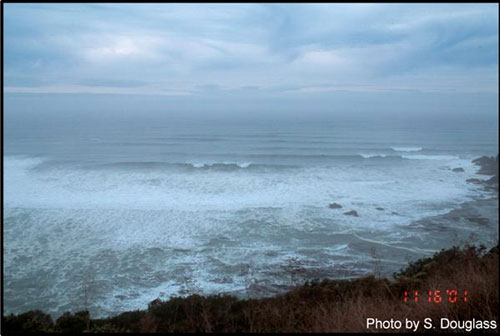Figure 4.11. A train of long-period swell approaching the coast.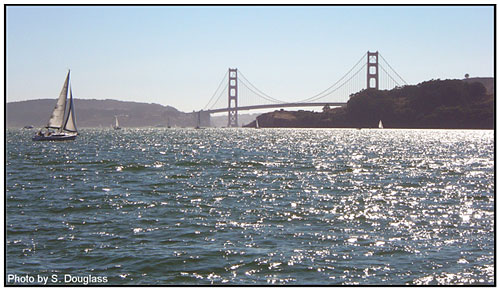Figure 4.12. Irregular waves on San Francisco Bay, California.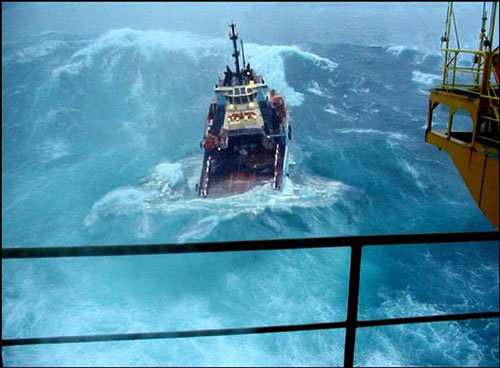Figure 4.13. A storm-driven, irregular, sea state.

“Significant wave height,” Hs, is a term with a long history of use in oceanography and coastal engineering. Two different sets of tools have been developed by oceanographers to describe realistic sea states and thus, significant wave height. One is a statistical representation of the individual wave heights in a sea state. This leads to a primary wave height definition called a “significant wave height”, the average height of the one-third highest waves. The other is a frequency spectrum representation of the water surface elevation that leads to a primary wave height definition that is also called the “significant wave height.” In the literature, the notation for the statistically-based Hs is often: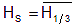(4.25)

and the notation used for the spectral significant wave height is: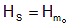(4.26)

The two definitions lead to values of significant wave height (Hs) that are approximately equal in deepwater seas. However, in shallow water, and especially in the surf zone, the two parameters diverge. The term “significant wave height” probably arose as a way for ship-board observers to estimate the wave height. Some argue that there is nothing truly “significant” about either definition since there are very few individual waves in an irregular sea that will be of the same height as the significant wave height. The significant wave height (Hs) for a sea state is a statistical artifact. However, Hs (with either definition) provides a consistent, meaningful measurement of the energy in a given sea state and thus most modern engineering methods use it.

Individual wave heights vary in an irregular sea state. The distribution of individual wave heights follows a Rayleigh probability distribution (see USACE 2002). This one-parameter distribution allows for estimation of other wave heights that are sometimes used in design. Table 4.1 provides the relation of some of these other wave heights to Hs. There are two types of statistics shown in Table 4.1:

• Those that are the average of waves with heights above a certain level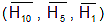• Those that are the wave height exceeded by a given percentage of waves in the irregular sea state (H10%, H1%).
Table 4.1. Wave height statistics in irregular seas.
Wave statistic Description Multiple of Hs =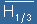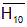average of the highest 10% of waves 1.27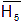average of the highest 5% of waves 1.38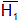average of the highest 1% of waves 1.67
H10% height exceeded by 10% of waves 1.07
H1% height exceeded by 1% of waves 1.52

Each of the wave transformations discussed above for the simpler monochromatic wave train model occur in irregular seas. This includes refraction, diffraction, shoaling, attenuation, and depth-limited breaking. A number of numerical approaches have been developed to model these wave transformations. This is an area of active research and rapid technology development.

##### 4.3.1 Numerical Models

One model for the transformation of irregular waves developed by the USACE is STWAVE. It has now been used on a number of engineering project studies (Smith and Smith, 2001). STWAVE is a finite-difference model designed to simulate the nearshore transformation of a directional spectrum of wave energy. A typical application is to take known offshore wave conditions, such as those measured by a NOAA buoy, and transform them over complex nearshore bathymetry, often to the point of nearshore breaking. Typical coverage areas are 10-20 km in the offshore direction and 20-40 km along the shore, with grid cell sizes ranging from 25 to 100 m. STWAVE is described in detail in Smith, et al. (2001).

#### 4.4 Wave Generation

Almost all water waves in the ocean and on bays are caused by winds. Wind first ripples the water surface and then begins to increase the heights of the ripples until they become small waves that propagate on their own. Wave heights continue to increase as the wind blows farther or harder across the water surface. If the water body is large enough, eventually the wave heights will stop growing unless the wind speed increases more. Once they are generated, waves often propagate for hundreds or thousands of miles across the ocean. They travel beyond the storm that generated them. Most waves that hit the shoreline were generated far out at sea. Waves that have traveled out of the winds that generated them are called “swell.” Waves that are still being acted upon by the winds that created them are called “sea.”

Fetch (F) is the distance across the water that a wind blows to generate waves. For enclosed bays, this is the maximum distance across the water body in the direction of the wind. Duration is the time that a wind blows. Waves are called “fetch-limited” if their height is limited by the available fetch distance. Waves are called “duration-limited” if their height is limited by the duration that the wind has blown. If winds blow long enough across a limited fetch until the sea state is no longer duration-limited, the sea is considered “fully-arisen.”

One of the products that came out of the World War II efforts to forecast surf and wave conditions for amphibious landings was an empirical method for estimating wave generation (Sverdrup and Munk 1947). That method was improved by Bretschnieder to form the method now known as the SMB method after those investigators. The USACE Shore Protection Manual (USACE, 1984) replaced the SMB method with a similar method based on more recent research in the JONSWAP experiments (Hasselmann, et al. 1980).

Appendix C presents a method to estimate wave height and period for shallow bays and lakes. The method has been placed in a spreadsheet model (Weggel 2005). A graph can then be plotted from the results helping to estimate the range of wave heights and periods at any specific location given the fetch and water depth. Figure 4.14 shows an example of such an analysis.

On the open ocean, waves are almost never fetch-limited and they continue to move after the wind ceases or changes. Swell wave energy can propagate very long distances and into other storms. Waves striking the shore at any moment in time may include swells from several different locations plus a local wind sea. Modern wave modeling can numerically solve wave generation and propagation equations using a grid across the entire ocean. These models can include wave generation as well as the transformations of refraction and depth-limited breaking. There are a number of available models including the Wave Analysis Model (WAM) (Komen, et al. 1994) and the Simulating Waves Nearshore (SWAN), (Booij, et al. 1999). This is an active area of research and the technology is still being developed and debated in the oceanography community. However, on a daily basis throughout the world, these and other models are used to forecast waves.

The same models used for wave forecasting can be used for wave “hindcasting.” Hindcasting is the application of the model to estimate wave conditions that occurred in the past. This can be done for historical storms or for long-term simulations. Figure 4.15 shows results from a hindcast of Hurricane Katrina using the SWAN model. The figure shows the maximum wave heights that occurred at each location in the immediate vicinity of the US 90 bridge across Biloxi Bay. The dashed line shows the bridge location. The colors refer to estimated significant wave heights for each location and the arrows indicate wave direction.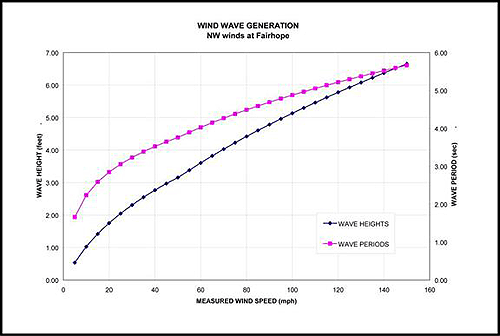Figure 4.14. Example of wave generation equations applied to a specific site.

The USACE Wave Information Study (WIS) has developed a hindcast database of wave conditions at hundreds of locations around the United States coastline. An ocean wave generation model (Resio 1981, Hubertz 1992) was used with 40 years of wind estimates generated from historical barometric pressure fields across the world. The results are estimates of wave conditions; Hs, Tp and wave direction; every 3 hours between 1956 and 1995.

The resulting data and summaries are available on-line at the USACE Coastal Hydraulics Laboratory web-site (//chl.erdc.usace.army.mil/chl/2006). These hindcast WIS data have been used to develop estimates of long-term wave statistics for engineering design and estimating longshore sand transport rates. Care should be taken in using these hindcast wave statistics for design since these results are not based on actual measurements but rather computer simulations.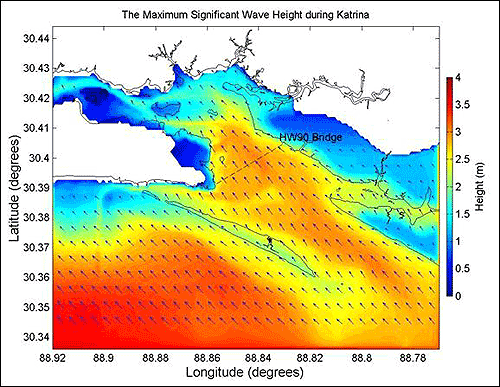Figure 4.15. Maximum significant wave heights generated during Hurricane Katrina in immediate vicinity of U.S. 90 bridge over Biloxi Bay as estimated by SWAN

#### 4.5 Tsunamis

Tsunamis are waves generated by underwater landslides caused by earthquakes. Tsunamis are the world’s most powerful water waves because they have extremely long wavelengths that transform significantly as they propagate into shallow water. Tsunamis are sometimes improperly called “tidal waves” even though they have nothing to do with the tides.

The “Boxing Day” tsunami of December 26, 2004 was one of the worst natural disasters of the past century. The runup from the tsunami around the Indian Ocean killed over 225,000 people and destroyed entire cities and villages. A tsunami that hit the Pacific coast of the United States in 1964 killed 12 people and caused millions of dollars in damages in northern California and Oregon. In 1946, a tsunami hit Hilo, Hawaii, killing 150 people.

The generation and propagation of tsunamis is an active area of oceanography research. The flow dynamics of a tsunami runup, including how it interacts with built infrastructure such as buildings and roads, can be very complex and is also an active area of civil engineering research. The destructive flows due to tsunami wave breaking and runup can vary greatly from location to location for the same tsunami based on local bathymetry and topography.

The Pacific coasts of the United States are clearly more susceptible to tsunami damage than the rest of the nation. Large portions of the United States Pacific coast and Hawaii have tsunami warning systems in place in recognition of the threat. However, tsunamis can occur along the Atlantic and Gulf coasts. The “Boxing Day” tsunami was generated in an area that had previously been thought to be unlikely for major tsunami generation.

While some State DOTs may have designed highways or bridges to account for potential tsunamis, the actual number and extent is not known. What is clear is that some portion of the transportation infrastructure, on all coasts, is clearly in the potential damage zone from tsunamis.

#### 4.6 Ship Wakes

Ship wakes are sometimes the largest waves that occur at sheltered locations and thus can be the design waves for revetments or other structures. Large ships can generate wakes with wave heights exceeding H=10 ft and smaller vessels (including tugboats) can generate wakes of H=5 ft.

The wake depends on the size, hull shape, speed of the vessel and distance from the sailing line. Engineering judgment based on observations can be used to establish a reasonable upper limit on wake size if the maximum speeds from all possible vessels are considered. Several methodologies for estimating ship wakes are available including Weggel and Sorensen (1986) and Kriebel, et al. (2003) for large vessels and Bottin, et al. (1993) for some smaller recreational watercraft.